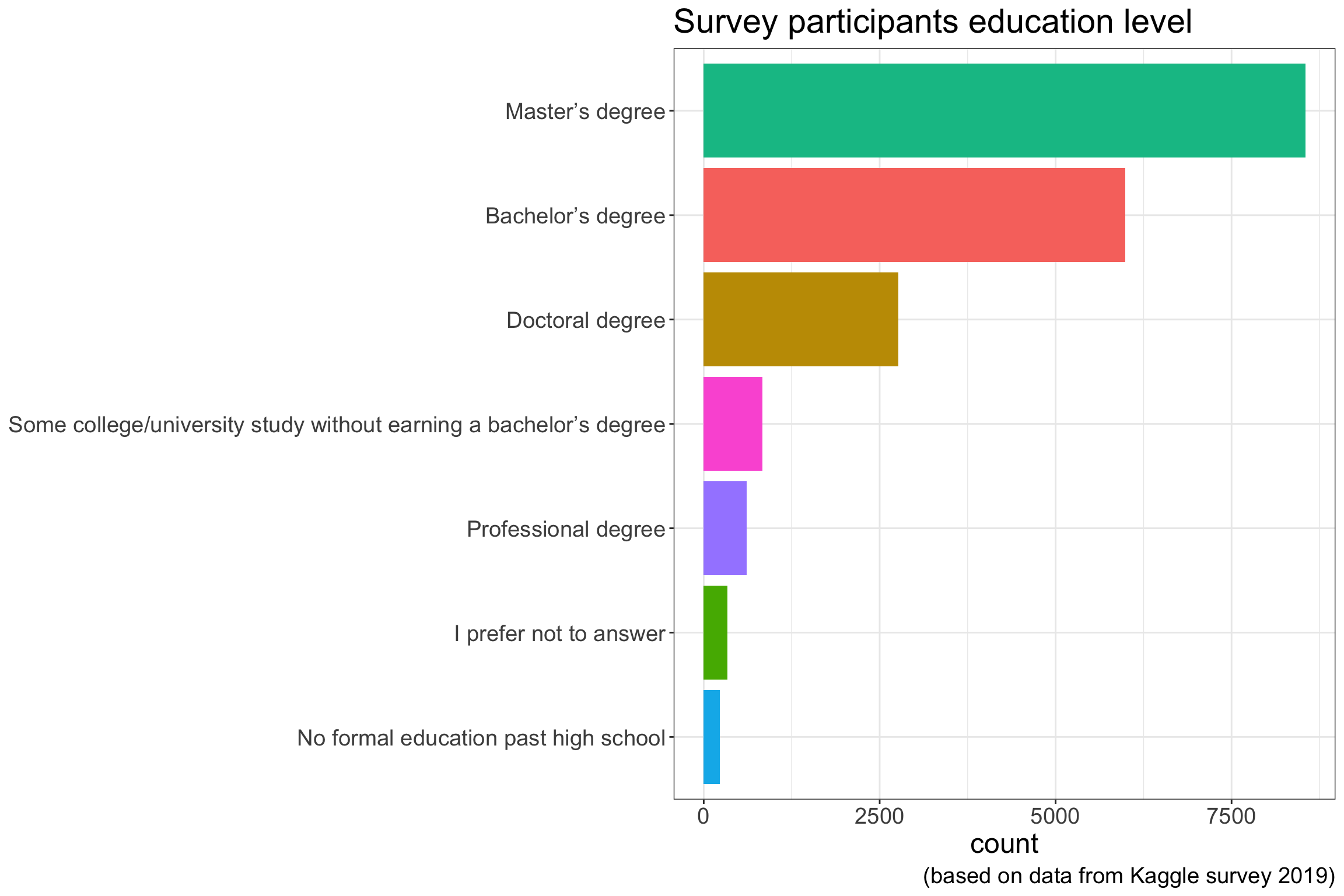## 27.1 Survey participants education level

The following plot shows survey participants education level. Very few participants have a non-academic background. By no means a academic background is a pre-requisit to use machine learning, however, two skills are very helpful

• Coding experience
• Statistical knowledge
• Youngest Kaggle Grandmaster at age of 16
• Mikel Bober-Irizar
• No formal education neededCoding experience speeds up the process to implement the machine learning ideas and concepts. Most effort during a machine learning project will go into

Main effort during ML process:

• Data pre-processing
• Model tuning

The actual implementation of the algorithm is often a matter of 10 - 20 lines of code. Below the neural network definition for a self driving RC model car of the donkey car framework.

The neural network is defined using the Keras API which sits on top of Tensorflow, the program is written in Python

    img_in = Input(shape=input_shape, name='img_in')
x = img_in
x = Convolution2D(24, (5,5), strides=(2,2), activation='relu', name="conv2d_1")(x)
x = Dropout(drop)(x)
x = Convolution2D(32, (5,5), strides=(2,2), activation='relu', name="conv2d_2")(x)
x = Dropout(drop)(x)
if input_shape > 32 :
x = Convolution2D(64, (5,5), strides=(2,2), activation='relu', name="conv2d_3")(x)
else:
x = Convolution2D(64, (3,3), strides=(1,1), activation='relu', name="conv2d_3")(x)
if input_shape > 64 :
x = Convolution2D(64, (3,3), strides=(2,2), activation='relu', name="conv2d_4")(x)
elif input_shape > 32 :
x = Convolution2D(64, (3,3), strides=(1,1), activation='relu', name="conv2d_4")(x)
x = Dropout(drop)(x)
x = Convolution2D(64, (3,3), strides=(1,1), activation='relu', name="conv2d_5")(x)

x = Flatten(name='flattened')(x)
x = Dense(100, activation='relu', name="fc_1")(x)
x = Dropout(drop)(x)
x = Dense(50, activation='relu', name="fc_2")(x)
x = Dropout(drop)(x)
angle_out = Dense(15, activation='softmax', name='angle_out')(x)
throttle_out = Dense(20, activation='softmax', name='throttle_out')(x)

model = Model(inputs=[img_in], outputs=[angle_out, throttle_out])